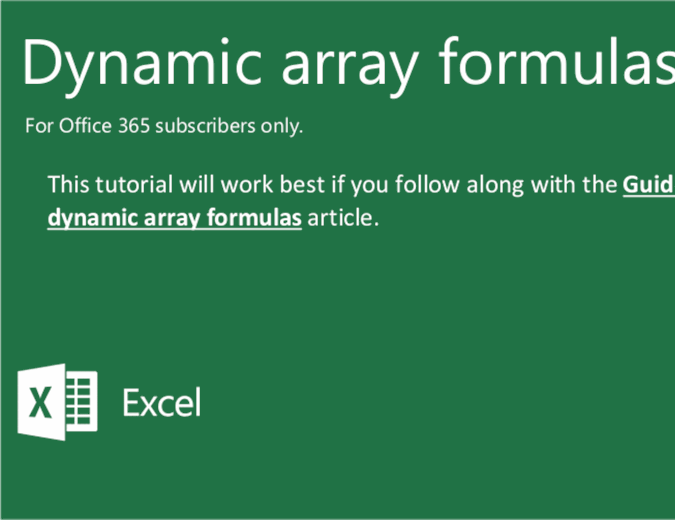# Dynamic array formulas tutorial

Become an Excel power user and learn about array formulas with the help of this tutorial. You can use array formulas to do complex tasks including calculate the total cost of a loan over any given number of years, count the number of characters that are contained in a range of cells, and much more.

Excel